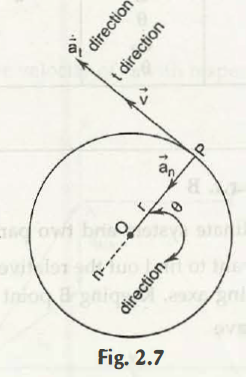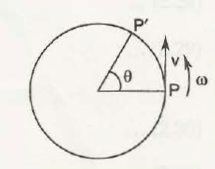# Special Case: Circular Motion

Boost your grades with best homework help from our qualified and skilled subject experts## Why Students Order Homework Help From Us### Last Minute Request

Our experts solve complex problems with high accuracy, ensuring fast, reliable help without compromise.### Custom Essay Writing

Professional essays for all subjects and degrees, guaranteed for accuracy, authenticity, and originality.### AI & Plagiarism-Free

Our skilled writers turn your ideas into 100% original, plagiarism- & AI-free papers.### Transparent Pricing

Suppose a particle is moving in a circular motion and we have to understand about its scalar components. In a generalized sense, those dual components are acceleration and velocity.
Let the 2 points of the straight line D and P be the half of the circular motion.
Let r, which is the radius of that circular path, be constant.
As per this information, calculations for both acceleration and velocity are:
v =r θ
an =v2/r = r θ 2 = v θ
at = dv/dt = v = rθ
Here, the measurement of ‘e’ is done from any side of convenient radial reference which then goes to DP.Angular Velocity
The rate of change of a rotating particle body in an angular position is known as the angular velocity of that particle.
Angular velocity is represented as w
Equation for angular velocity is,
W = dθ/dt
According to another figure of a particle moving in a circular motion, its linear velocity is represented as,
Linear velocity= r x angular velocity
The next equation stands at, v rθ = rw
Unit of angular velocity (w) = rad /sAngular Acceleration
The rate of change of angular velocity is known as angular acceleration.
Its equational definition is,
a = dw/dt = d2θ/dt2 = θ = w
Equation for tangential equation is,
at = dv/dt = v = rθ
This calculation further goes to,
= r w = r dw/dt
= ra
In case of normal acceleration, its equation is,
v2/r = w2r2/r = w2r
Derivational equations for linear velocity are applicable for angular velocity.
If a certain instance is taken, equation for angular velocity is,
w = w0 +a tà
Equation for linear velocity is,
v = v0 +atà